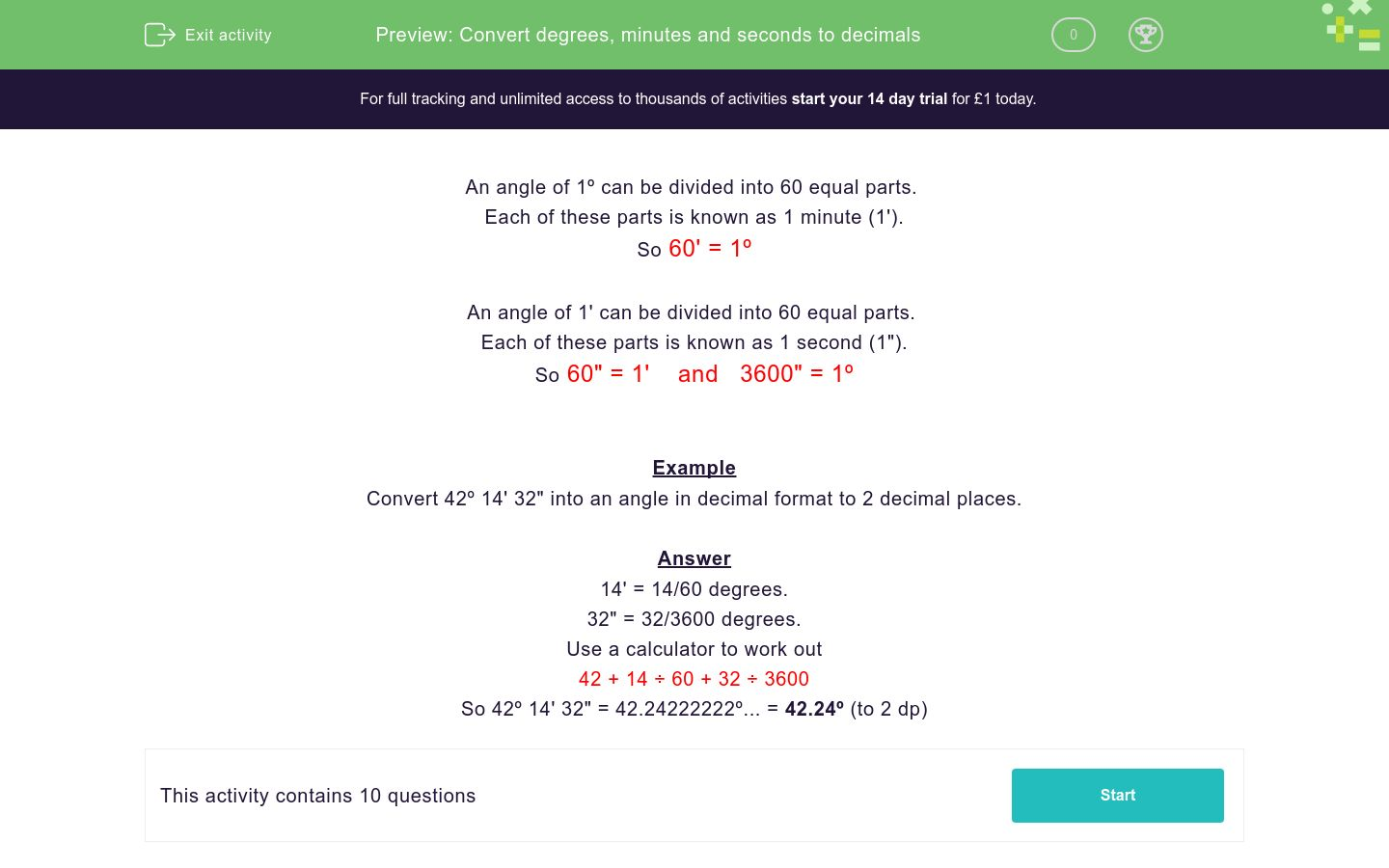# Convert degrees, minutes and seconds to decimals

In this worksheet, students use a calculator to convert angles given in degrees, minutes and seconds to angles in decimal format.Key stage:  KS 2

Curriculum topic:   Number: Addition, Subtraction, Multiplication and Division

Curriculum subtopic:   Solve Four Operations Problems

Difficulty level:#### Worksheet Overview

An angle of 1º can be divided into 60 equal parts.

Each of these parts is known as 1 minute (1').

So 60' = 1º

An angle of 1' can be divided into 60 equal parts.

Each of these parts is known as 1 second (1").

So 60" = 1'    and   3600" = 1º

Example

Convert 42º 14' 32" into an angle in decimal format to 2 decimal places.

14' = 14/60 degrees.

32" = 32/3600 degrees.

Use a calculator to work out

42 + 14 ÷ 60 + 32 ÷ 3600

So 42º 14' 32" = 42.24222222º... = 42.24º (to 2 dp)

### What is EdPlace?

We're your National Curriculum aligned online education content provider helping each child succeed in English, maths and science from year 1 to GCSE. With an EdPlace account you’ll be able to track and measure progress, helping each child achieve their best. We build confidence and attainment by personalising each child’s learning at a level that suits them.

Get started